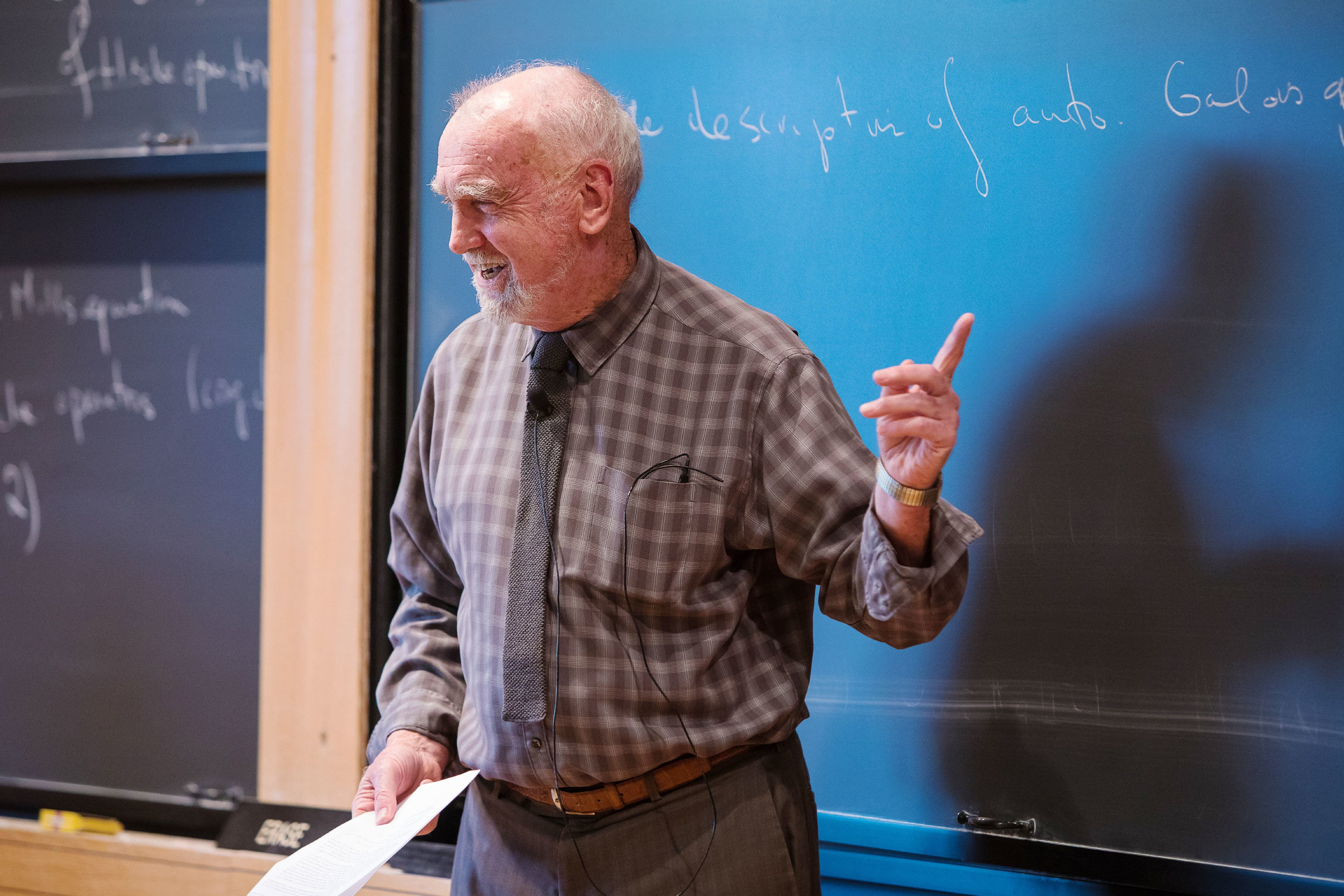# Robert Langlands, Mathematical Visionary, Wins the Abel Prize

Generations of researchers have pursued his “Langlands program,” which seeks to create a grand unified theory of mathematics.Explore

Robert Langlands, who developed one of the most original insights of 20th-century mathematics, was named the winner of the 2018 Abel Prize at a ceremony in Norway this morning. The prize, which is modeled on the Nobel, is one of the highest honors in mathematics.

Langlands, 81, an emeritus professor at the Institute for Advanced Study in Princeton, New Jersey, is the progenitor of the “Langlands program,” which explores a deep connection between two pillars of modern mathematics: number theory, which studies arithmetic relationships between numbers, and analysis, which is an advanced form of calculus. The link has far-reaching consequences that mathematicians have used to answer centuries-old questions about the properties of prime numbers.

Langlands first articulated his vision for the program in 1967—when he was 30—in a letter to the famed mathematician André Weil. He opened the 17-page missive with a now-legendary stroke of modesty: “If you are willing to read it as pure speculation, I would appreciate that,” he wrote. “If not—I am sure you have a waste basket handy.”

Since then, generations of mathematicians have taken up and expanded upon his vision. The Langlands program now ranges over so many different fields that it is often referred to as the search for a “grand unified theory” of mathematics.

“It’s revolutionary, I think, as far as the history of mathematics is concerned,” said James Arthur, a mathematician at the University of Toronto and former student of Langlands.’

Mathematicians have always been interested in finding patterns in prime numbers—those numbers that are divisible only by one and themselves. Primes are like the atomic elements of number theory, the fundamental pieces from which the study of arithmetic is built. There are an infinite number of them, and they appear to be scattered randomly among all numbers. To find patterns in primes—like how frequently they occur (which is the subject of the famous Riemann hypothesis)—it’s necessary to relate them to something else. Seen correctly, the primes act like a cipher, which turns into a beautiful message when read through the right key.

“They look like random accidents, but especially through the Langlands program, it’s turning out they have an extremely complex structure that relates them to all sorts of other things,” said Arthur.

One question about the structure of primes is which primes can be expressed as a sum of two squares. The first few examples include:

5, a prime number that equals 22 + 12,

13, which equals 32 + 22, and

29, which equals 52 + 22.

In the 17th century, number theorists discovered that all primes that can be expressed as a sum of two squares share another property: They leave a remainder of 1 when divided by 4. The work began to reveal a hidden structure to the primes. Then in the late 18th century, Carl Friedrich Gauss generalized this surprising link, formulating a “reciprocity” law that linked certain primes (those that are a sum of two squares) to an identifying characteristic (when divided by 4, they leave a remainder of 1).

In his letter, Langlands proposed a vast extension of the kind of reciprocity law Gauss had discovered. Gauss’s work applied to quadratic equations—those with exponents no higher than the number 2. Langlands suggested that the prime numbers encoded in higher-degree equations (like cubic and quartic equations) should be in a reciprocity relationship with the far-off mathematical land of harmonic analysis, which grows out of calculus and is frequently used to solve problems in physics.

For example, scientists in the 19th century were surprised to discover that when they looked at starlight through a prism, they didn’t find a continuous spectrum of colors. Instead, the spectrum was interrupted here and there by black lines, now called absorption spectra, where the light was missing. Eventually the scientists realized that the missing light had been absorbed by elements in the stars. This discovery provided solid evidence that the stars and our planet are made from the same material.

At the same time, the spectral lines became objects of mathematical interest. The missing wavelengths gave a sequence of numbers—the frequencies of the absent light. Mathematicians could study those numbers through analysis. Or they could work on wholly new kinds of equations—inspired, perhaps, by questions in physics, but arising purely from analysis and geometry. Based on those new equations, they could study a parallel notion of absorption spectra.

The Langlands program relates prime number values of polynomial equations to spectra from the differential equations studied in analysis and geometry. It says that there should be a reciprocity relationship between the two. As a result, you should be able to characterize which prime numbers appear in specific settings by understanding which numbers appear in the corresponding spectra.

The two sets of numbers can’t be compared directly, though. They each have to be translated through different kinds of mathematical objects. In particular, Galois representations, which are based on primes, should pair with objects called automorphic forms, which contain the relevant spectra.

Today mathematicians working in the Langlands program are trying to prove that relationship and many other related conjectures. At the same time, they’re using Langlands-type connections to solve problems that would otherwise seem out of reach. The most celebrated result in this regard is Andrew Wiles’s proof in 1995 of Fermat’s Last Theorem. Wiles’s proof depended in part on exactly the type of relationship between number theory and analysis that Langlands had predicted decades earlier.

The Langlands program has expanded considerably over the years. Yet when you push aside all the complex machinery that’s been created to realize Langlands’ vision, you see that the whole massive enterprise remains motivated by some of the most basic of mathematical concerns.

“Understanding the properties of which primes occur in an equation basically amounts to a fundamental classification of the arithmetic world,” said Arthur.